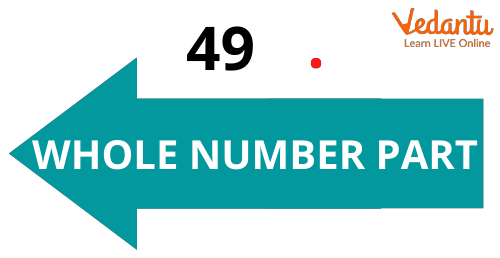Courses
Courses for Kids
Free study material
Free LIVE classes
More

# All About Decimal Point and Decimal Number## What is a Decimal Point?

Before understanding decimal points we should know about decimal numbers. So, the decimal number is a number that is made up of the whole number part and fraction part. The decimal is in the form of a point that is used to separate the part of a whole number from the fractional part of a decimal number. Hence, these are numbers in between whole numbers. An example of a decimal number is 15.2, which is between 15 and 16. It is more than 15, but less than 16. Decimal numbers are the same as fractions but their method of expression is different. One more example of a decimal number is given below.Example of Decimal Number

## Points to Remember

A few points are mentioned below that are necessary to remember.

• If 49.15 is a decimal number and you are confused about which part is a whole number and which is a rational number, remember that the whole number is written to the left of the decimal point.Whole Number

The fractional part in a decimal number is usually written to the right of the decimal point.Fractional Number

• The use of decimal points makes the reading of decimal numbers easy. A decimal number can be read in two ways. They are as follows.

(i) Reading decimal points as “and”:

In this method, the whole number part is read as it is and the decimal point is read as “and”. The fractional part is then read. For example, the number 363.16 is read as “three hundred sixty-three and sixteen hundredths”.

(ii) Reading the decimal point as “point”:

In this method, the whole number part is read as it is and the decimal point is read as “point”. Then the fractional part to the right of the decimal point is read as individual digits. For example, the number 363.16 is read as “three hundred sixty-three point one six”.

## Tips for Teaching Decimal to Kids

• Before starting any new concept, it is important that kids revise previous concepts. When teaching decimal numbers, first revisit and revise the basics of thousands, hundreds, tens and ones and then introduce or revise the tenths, hundredths and thousandths.

• You can do activities with money and explain how the coins are related to ones, tenths and hundredths.

## Do You Know?

• In a decimal number, the fractional part is present to the right of the decimal point, which denotes a number less than 1.

• A whole number can also be written as a decimal number by writing .0 (point zero) after the one digit. For example, we can write 34 as 34.0.

• The decimal point is also referred to as a decimal separator.

## Conclusion

When kids move to higher classes, the concept of decimal numbers becomes very essential for them to solve the problem. In this article, the decimal point is explained through examples that would help students to grab the concept of decimal numbers.

Last updated date: 18th Sep 2023
Total views: 126.3k
Views today: 2.26k

## FAQs on All About Decimal Point and Decimal Number

1. How do you explain a decimal to a child?

You can teach decimal numbers to your child by giving examples and clearing their doubts. Before explaining decimal numbers,  concepts such as whole numbers, fractional numbers, tens, ones, etc. should be taught or revised.

2. What is the use of decimal points?

The use of decimal points in decimal numbers is to separate the whole number and the fractional number. On seeing a decimal point in a number, we can identify that it is a decimal number.